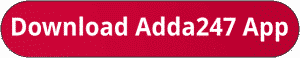Latest Banking jobs   »   sbi quant

# Quantitative Aptitude Quiz For SBI PO/Clerk Prelims 2023 -19th October

Directions (1-5): Read the following bar graph and table carefully and answer the questions given below. Bar graph shows percentage distribution of total number of vehicles (bikes and cars) in five different cities (P, Q, R, S & T) and table shows ratio of number of cars to bikes in these cities.Q2. If number of buses in T is 75% of number of bikes in R, then find the ratio of number of cars in Q to number of buses in T.
(a) 32 :15
(b) 32 :17
(c) 34 :19
(d) 31 :16
(e) 36 :17

Q3. If number of cars in A is 25% less than number of bikes in Q and ratio of number of bikes in A to cars in P is 7:5 respectively, then find the total number of vehicles (cars + bikes) in A?
(a) 1250
(b) 1800
(c) 2050
(d) 1500
(e) 1450

Q4. Find the difference between number of bikes in R and average number of cars in T and S?
(a) 45
(b) 20
(c) 50
(d) 65
(e) 30

Q5. If ratio of SUV cars and Sedan cars in P & S is 5: 7 and 1: 2 respectively, then total SUV cars in P and S together is how many more/less than total Sedan cars in P and S together (Note: Total cars in any city = SUV cars + Sedan cars)?
(a) 150
(b) 250
(c) 180
(d) 125
(e) 175

Directions (6-10) :- What approximate value should come in place of question mark (?) in following questions.

Q6. 17.97% of 649.90 – 8.02% of 1149.99 = ?²
(a) 4
(b) 5
(c) 3
(d) 6
(e) 7Q8. 30.08% of 4/7 of 1/8 of 419.91 = ?
(a) 6
(b) 18
(c) 15
(d) 12
(e) 9

Q9. 719.97 ÷ 80.02 ÷ 60.07 × 119.97 = ?
(a) 14
(b) 20
(c) 18
(d) 10
(e) 24

Q10. 899.9 × 25.02 ÷ 35.99 = (? + 17.03)²
(a) 10
(b) 5
(c) 18
(d) 12
(e) 8

Solutions. .               .                                      .                       .                                         .

## FAQs

### What sections are included in the SBI PO or SBI Clerk Prelims Exam?

The selection process of SBI Clerk 2023 consists of Prelims, Mains and LPT.

#### Congratulations!Union Budget 2023-24: Free PDF